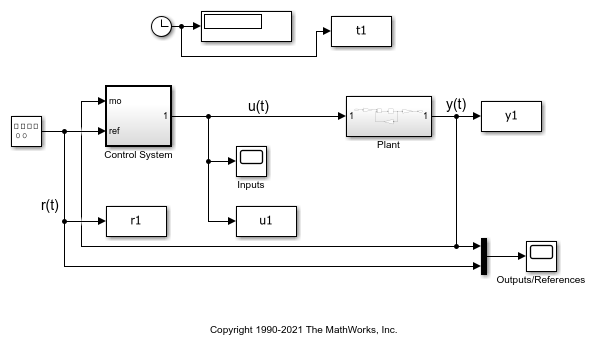# Simulation and Structured Text Generation for MPC Controller Block

This example shows how to simulate and generate structured text for an MPC Controller block by using Simulink® PLC Coder™ software. The generated code uses single-precision data.

### Required Products

To run this example, Model Predictive Control Toolbox™, Simulink®, and Simulink PLC Coder™ are required.

```if ~mpcchecktoolboxinstalled('simulink') disp('Simulink is required to run this example.') return end if ~mpcchecktoolboxinstalled('plccoder') disp('Simulink PLC Coder is required to run this example.'); return end if ~mpcchecktoolboxinstalled('mpc') disp('MPC Toolbox is required to run this example.'); return end ```

### Define Plant Model and MPC Controller

Define a SISO plant.

```plant = ss(tf([3 1],[1 0.6 1])); ```

Define the MPC controller for the plant.

```Ts = 0.1; %Sample time p = 10; %Prediction horizon m = 2; %Control horizon Weights = struct('MV',0,'MVRate',0.01,'OV',1); % Weights MV = struct('Min',-Inf,'Max',Inf,'RateMin',-100,'RateMax',100); % Input constraints OV = struct('Min',-2,'Max',2); % Output constraints mpcobj = mpc(plant,Ts,p,m,Weights,MV,OV); ```

### Simulate and Generate Structured Text

```mdl = 'mpc_plcdemo'; open_system(mdl) ```To generate structured text for the MPC Controller block, put the MPC block inside a subsystem block and treat the subsystem block as an atomic unit. Select the Treat as atomic unit property of the subsystem block.

```close_system([mdl '/Control System/MPC Controller']) open_system([mdl '/Outputs//References']) open_system([mdl '/Inputs']) sim(mdl) ```
```-->Converting model to discrete time. -->Assuming output disturbance added to measured output channel #1 is integrated white noise. -->The "Model.Noise" property is empty. Assuming white noise on each measured output. ```To generate code by using the Simulink PLC Coder, use the `plcgeneratecode` command.

```disp('Generating PLC structure text... Please wait until it finishes.') % % plcgeneratecode([mdl '/Control System']); ```
```Generating PLC structure text... Please wait until it finishes. ```

The Message Viewer dialog box shows that PLC code generation was successful.

```bdclose(mdl) ```